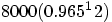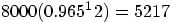Philip's Content

There have been 13 items by Philip (Search limited from 19-January 21)

See this member's

Sort by                Order

#102262Quick questionPosted by on 31 May 2009 - 09:45 AM in Geography

QUOTE (jamsand @ May 31 2009, 10:17 AM) <{POST_SNAPBACK}>
I want to know what happens if your slightly loopy like me and answer both question 5 and 6 do they disregard the highest one or just mark the first one they come across? any insight is much appreciated:)

They mark the first one. Let's hope you did well in question 5#1022352009 Higher PhysicsPosted by on 26 May 2009 - 06:06 PM in Physics

I thought the paper was quite fair. Not too many overly complicated questions. Though I did find question 21 a little harder than the rest - or maybe that's just me!How did everyone else do?

#102234Wrote in pencilPosted by on 26 May 2009 - 06:00 PM in Physics

QUOTE (Christer @ May 26 2009, 05:25 PM) <{POST_SNAPBACK}>
I finished my physics exam and realised I had written everything in pencil, would I be failed because of this? It said at the start of the paper to write in pen (wish I'd read that)!

They might be lenient on youBut, you never know cos a friend of mine failed his Product Design prelim cos he wrote in pencil!
Let's just hope their fair...#102219One Quick Question...Posted by on 23 May 2009 - 07:18 PM in Mathematics

QUOTE (laura_lou92 @ May 20 2009, 11:15 PM) <{POST_SNAPBACK}>
Use pencil for objective questions and pen for the rest.
well thats what iv always been told =) good luck!Thanks, i took your advice. Loads of my friends at school got a right bollocking from the chief invigilator cos they didn't have a pencil for the objective test!#102170One Quick Question...Posted by on 20 May 2009 - 08:12 PM in Mathematics

Do we use pen or pencil for the exam? Or both?I know it sounds stupid but i can't remember which one your allowed to use#102158Paper 2 2005Posted by on 20 May 2009 - 03:15 PM in Mathematics

QUOTE (laura_lou92 @ May 20 2009, 03:52 PM) <{POST_SNAPBACK}>
not entirely sure what to do... anyone thing they could help?

log4(5-x) - log4(3-x) = 2 x<3

Haha i just did this one 5 minutes agoSo you re-arrange to log_{4}(5-x)-log_{4}(3-x)=log_{4}4^2 Remember : log_{4}4=1

Then you can cancel out the logs:

(5-x)/(3-x)=4^2

16(3-x)=(5-x)

48-16x=5-x

5-15x=48

-15x=43

x=-43/15

Hope this helped! Any questions just reply back.

#1021542006 Paper 2 Question 12 bPosted by on 20 May 2009 - 01:26 PM in Mathematics

QUOTE (Garden @ May 20 2009, 12:24 PM) <{POST_SNAPBACK}>
QUOTE (Philip @ May 20 2009, 11:43 AM) <{POST_SNAPBACK}>
Need some help with the 2006 Paper 2 Question 12 b

Thanks!Handily you can find the HSN worked solution at Google Books but here's how I would do it (not too different really)...

Remember that extremes (that is the mins and maxes) can occur at stationary points or the start and end of the given range (underlined as that's probably what you've done wrong)

So, A = 80 - 12x - 48^-1
dA/dx = -12 + 48x^-2 = 0 (for stat. point)
-12 + 48/x^2 = 0
48/x^2 = 12
12x^2 = 48
x^2 = 4
x = 2 or -2
Thus x = 2 as x > 0.

So find A for the three values of x...
When x = 1, A = 80 - 12x1 - 48/1^2 = 20
When x = 2, A = 80 - 12x2 - 48/2^2 = 32
When x = 4, A = 80 - 12x4 - 48/4^2 = 20

Therefore: Max of A is 32 when x = 2 and Min of A is 20 when x = 1 or 4

For the three values at the end: did you just use the x=1 and x=4 from the graph to find the mins and maxs?

What i did wrong was that i found the minimum x=2, but didn't know you just subbed in the other two values.

Thanks for the help#1021472006 Paper 2 Question 12 bPosted by on 20 May 2009 - 10:43 AM in Mathematics

Need some help with the 2006 Paper 2 Question 12 b

Thanks!#1021462007 Past Paper QuestionPosted by on 20 May 2009 - 10:38 AM in Mathematics

QUOTE (Ailso @ May 18 2009, 01:53 PM) <{POST_SNAPBACK}>
This is how i got it...not sure its the correct way though, but i got the answer. (Sorry if its messy dont know how to do the shortcut things on this site! Also, i dont know where the marks will be allocaed either.)

1) Work out point B to be (7,8)

2) Radius of the large circle = square root of ((g squared) +(f squared) - c)

i.e,square root of ((7squared)+(8squared)-77) = square root of 36 = 6

therefore radius of large circle = 6

3) Between the point B and the outer of the circle there are three sets of radii this means that the radii of the smaller
circles is the radius of the large circle divided by three, i.e 6 divided by 3=2, this is the length of each of the small radii
because the question tells us they are congruent.

4) The y-coordinate is the same for each of the centres cause they are parallel to the x-axis. So for D y=8

5) To get the x-coordinate for D use the x-coordinate from B. So it would be x-coordinate + radius of large circle + radius
of small circle, i.e 7+6+2=15.

6) The point D has coordinates (15,8)

7) Put these numbers into the equation of a circle ((x-a)squared + (y-b)squared = radius squared)to give us:

(x-15)squared + (y-8)squared = 2squared

Hope this helps, if you need anymore explanation, just ask!Thanks!#1021112007 Past Paper QuestionPosted by on 18 May 2009 - 12:09 PM in Mathematics

Just been doin a few past paper questions and got a little stuck...2007 Paper 1 Question 5

I get the centre of the circle. But i don't know what to do from there!

Would appreciate some help, thanks#102028Anyone do it?....Posted by on 03 May 2009 - 06:14 PM in Spanish

Yeah I'm doin Higher Spanish this year. Personally, i find it quite easy and hope to get the exam over with.Did Standard Grade Spanish and got a 1 so i should be ok#101880Recurrence RelationsPosted by on 08 April 2009 - 01:30 PM in Mathematics

QUOTE (Karennm @ Apr 7 2009, 03:28 PM) <{POST_SNAPBACK}>
The monks of St. Columbas Priory distil their own whisky. The whisky is matured for 12 years in oaks casks, during which time it loses 3.5% of its volume each year due to evaporation.
The monks prepare 8000litres of spirit estimating that this will produce at least 4000litres of whisky in 12 years time. Are they right?Hope i get this right...Ok, so we know that they prepare 8000 litres of spirit. And each year it loses 3.5% of volume; or you could look at it as having 96.5% of its original volume (which is probably easier here). And the time is 12 years.

This is just a depreciation question.

Therefore the numbers we have taken out of the question can be put like this:(Don't know why but the 2 of '12' doesn't want to be powered, just imagine it is)

Firstly, we have the number being inputted - 8000. Then we just multiply it by the 96.5% or 0.965 - as 1.0 would be 100%; 0.5 would be 50%. This number is the 'powered' by the number of years in question - in this case it's 12.

So all that is left to do is put the number into the equation, to get:Once you have written that, you write a conclusion saying: "After 12 years there is 5217 litres left. As 5217>4000, the monks are correct."

Hope this helped!

#101773HSN Notes for Higher PhysicsPosted by on 14 February 2009 - 04:05 PM in Physics

Is it possible that you could get the rest of unit 1 finished soon...and maybe 2/3 as well? I'd really appreciate it. These are great revision materials.